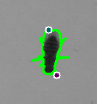# Datasets¶

The datasets contain properties of an object. They are added to an object by some modules.

For example, the base properties of a path or a contour are added by the “Track objects” module. The color average property is added by the module “Extract the contours’ images”.

## Path¶Property Sub-property Description Provided by the module
position
x X coordinate
y Y coordinate
velocity
x X velocity component
y Y velocity component
absolute sqrt(X*X+Y*Y)
acceleration
x X velocity component
y Y velocity component
absolute sqrt(X*X+Y*Y)
color average     Extract the contours’ images
red Average red color on a circle around each position of the path.
gree Average green color on a circle around each position of the path.
blue Average blue color on a circle around each position of the path.
motion   Number of pixels changed on a circle around each position of the path. Motion

## Contours¶Property Sub-property Description Provided by the module
position
x X coordinate
y Y coordinate
velocity
x X velocity component
y Y velocity component
absolute sqrt(X*X+Y*Y)
acceleration
x X velocity component
y Y velocity component
absolute sqrt(X*X+Y*Y)
color average     Extract the contour’s images
red Average red color on a circle around each position of the path.
gree Average green color on a circle around each position of the path.
blue Average blue color on a circle around each position of the path.
motion   Number of pixels changed on a circle around each position of the path. Motion
area   Contour area
perimeter   Contour perimeter
equivalent diameter   = sqrt(4*area/PI)
bounding rect   Result of the OpenCV function cv2.boundingRect
left x
left y
width
height
aspect ratio = (bounding rect > width) / (bounding rect > height)
area
perimeter = 2*(bounding rect > width) + 2*(bounding rect > height)
equivalent diameter = sqrt(4* (bounding rect > area) /PI)
extend = area / (bounding rect > area)
fit ellipse   result of the OpenCV function cv2.fitEllipse
center x
center y
major axis size
minor axis size
angle
extreme points   The 2 furthest points to the center
p1
p1 > x
p1 > y
p2
p2 > x
p2 > y
angle
convex hull
area
perimeter
equivalent diameter = sqrt( 4* (convex hull > area) /PI)
solidity = area / (convex hull > area)
rotated rectangle   result of the OpenCV function cv2.minAreaRect
center x
center y
width
height
angle
minimum enclosing circle   result of the OpenCV function cv2.minEnclosingCircle
x
y
minimum enclosing triangle   result of the OpenCV function cv2.minEnclosingTriangle
p1
p1 > x
p1 > y
p2
p2 > x
p2 > y
p3
p3 > x
p3 > y
perimeter = dist(p1, p2) + dist(p2, p3) + dist(p3, p1)
moments
m00, ..., m03, mu20, ..., mu03, nu20, ..., nu03 All of the moments up to the third order of a polygon or rasterized shape. Result of the function cv2.moments from the OpenCV library.
hu moments
hu, ..., hu The seven Hu invariant given by the function cv2.HuMoments from the OpenCV library